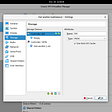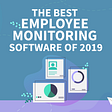# Leetcode

39. Combination Sum

Given an array of distinct integers `candidates` and a target integer `target`, return a list of all unique combinations of `candidates` where the chosen numbers sum to `target`. You may return the combinations in any order.

The same number may be chosen from `candidates` an unlimited number of times. Two combinations are unique if the frequency of at least one of the chosen numbers is different.

It is guaranteed that the number of unique combinations that sum up to `target` is less than `150` combinations for the given input.

Example 1:

`Input: candidates = [2,3,6,7], target = 7Output: [[2,2,3],]Explanation:2 and 3 are candidates, and 2 + 2 + 3 = 7. Note that 2 can be used multiple times.7 is a candidate, and 7 = 7.These are the only two combinations.`

Example 2:

`Input: candidates = [2,3,5], target = 8Output: [[2,2,2,2],[2,3,3],[3,5]]`

Example 3:

`Input: candidates = , target = 1Output: []`

Example 4:

`Input: candidates = , target = 1Output: []`

Example 5:

`Input: candidates = , target = 2Output: [[1,1]]`

Constraints:

• `1 <= candidates.length <= 30`
• `1 <= candidates[i] <= 200`
• All elements of `candidates` are distinct.
• `1 <= target <= 500`

Solution(Python3):

`class Solution:    def combinationSum(self, candidates, target):        ret = []        self.dfs(candidates, target, [], ret)        return ret    def dfs(self, nums, target, path, ret):        if target < 0:            return        if target == 0:            ret.append(path)            return        for i in range(len(nums)):            self.dfs(nums[i:], target - nums[i], path+[nums[i]], ret)# TC: O(N^(T/M)+1), where N is the number of candidates, T is the target value, and M is the minimum value among the candidates# SC: O(T/M)`

--

--

--

## More from JEN-LI CHEN IN DATA SCIENCE

My homepage to record my thought processes for solving SQL and Algorithm questions

## 🐎Griffin Race — ELO Calibration matches## Monitor SSH Session Connection Attempts using Grafana, InfluxDB and Telegraf## 4 Ways to get the most from an iOS Coding Bootcamp## Design Patterns — overview## How To Choose Right DevOps Tools For Your Business | HData Systems## The Best Employee Monitoring Software of 2019## Isabelle

In love with telling stories with data